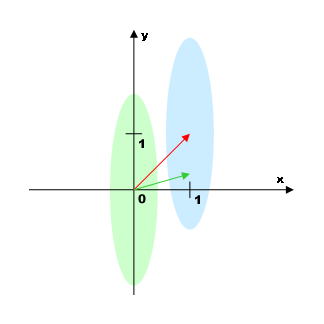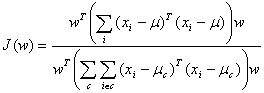# Linear discriminant analysis

Linear Discriminant Analysis (LDA) is a method of finding such a linear combination of variables which best separates two or more classes. In itself LDA is not a classification algorithm, although it makes use of class labels. However, the LDA result is mostly used as part of a linear classifier. The other alternative use is making a dimension reduction before using nonlinear classification algorithms.

It should be noted that several similar techniques (differing in requirements to the sample) go together under the general name of Linear Discriminant Analysis. Described below is one of these techniques with only two requirements: (1) The sample size shall exceed the number of variables, and (2) Classes may overlap, but their centers shall be distant from each other.

# Fisher's Linear Discriminant AnalysisThe figure on the right side shows an example of a problem solved using the Fisher algorithm.

There are two classes in the two-dimensional space of independent variables. The areas occupied by the classes take the form of long parallel ellipses (marked in green and blue). The center of the first class is positioned in the point (0, 0), and the second-class center is in the point (1, 1).

The simplest solution, that is, to draw a straight line connecting centroids of the classes (red arrow) and to project the points on it, would be inappropriate, as in that case the classes should overlap each other. Fisher's contribution is to find such an axis that projecting thereon should maximize the value J(w), which is the ratio of total sample variance to the sum of variances within separate classes:(where the summation over c means summing within classes, ν is a sample mean, ν is a group mean for Class c). As it is intuitively perceptible, images are best separated when each individual class have a minimum variance, with a maximum sampling variance in large. Thus, the optimum linear separation task is reduced to the problem of making a search for the minimum of a function J(w). Due to the numerator's and denominator's linearity, this problem may be brought to searching eigenvalues of a symmetric matrix.

The result is the N-dimensional vector which maximizes J(w), as well as N-1 of additional vectors forming the full basis, together with the first-mentioned one, and being ordered in the J(w) descending sequence (these vectors can be used, if we need find not just one axis but a subspace minimizing J(w)). The vector corresponding to the maximum of J(w) in the example above is represented in green. The maximum of information is apparently given by the first component of the vector, while second component may provide some useful information too. In reality, however, the angle between the vector and the axis x is even smaller, that is, in practice, classes are separated using the first component only.

# Applicability Limits

It may be noted that the classes do not overlap each other in the above example, but in a number of cases, the algorithm can be successfully used with overlapping classes as well. Class boundary overlapping is of little importance, so long as their centroids are distant from each other enough for variance to be changed substantially, depending on whether we calculate it as per the sample as a whole or within the classes. In an extreme case, when the centers of classes are coincident, that is, ν = ν, the numerator and denominator of J(w) will coincide, and the whole fraction will be identically equal to 1. In practice, that will be shown by the "wobbling" of the vector w pointing to different sides, depending on random correlations that will arise due to the finite size of the sample.

# Download ALGLIB for C++ / C# / ...

ALGLIB Project offers you two editions of ALGLIB:

ALGLIB Free Edition:delivered for freeoffers full set of numerical functionalityextensive algorithmic optimizationsno low level optimizationsnon-commercial license

ALGLIB Commercial Edition:flexible pricingoffers full set of numerical functionalityextensive algorithmic optimizationshigh performance (SMP, SIMD)commercial license with support plan

## ALGLIB 3.15.0 for C++C++ library.
Delivered with sources.
Monolithic design.
Extreme portability.
Editions:   FREE   COMMERCIAL

## ALGLIB 3.15.0 for C#C# library with native kernels.
Delivered with sources.
VB.NET and IronPython wrappers.
Extreme portability.
Editions:   FREE   COMMERCIAL

## ALGLIB 3.15.0 for DelphiDelphi wrapper around C core.
Delivered as precompiled binary.
Compatible with FreePascal.
Editions:   FREE   COMMERCIAL

## ALGLIB 3.15.0 for CPythonCPython wrapper around C core.
Delivered as precompiled binary.
Editions:   FREE   COMMERCIAL